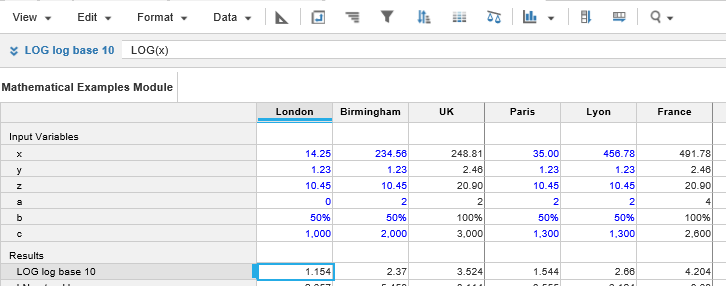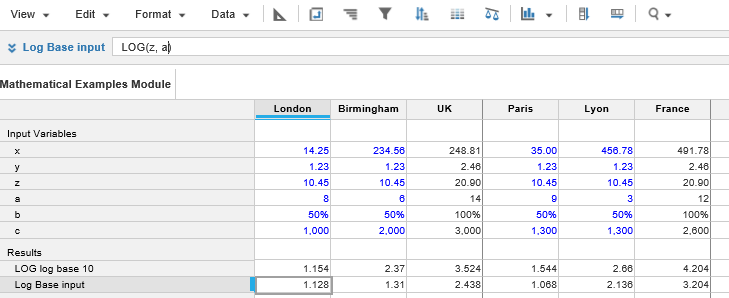1. Calculation functions
2. All Functions
3. Numeric Functions
4. LOGReturns the logarithm of a number to the specified base.

This function is the inverse of the POWER function.

## Syntax

`LOG(x,n) `

where:

• x: Number: Number to evaluate
• n: Number: Base to apply

LOG(x) is also valid with base 10 as default.

## Format

Input Format Output Format

x: Number

n: Number

Number

## Arguments

The function uses the following arguments:

• x: Number: Numeric line item, property, or expression.
• n: Number: Hard-coded number, numeric line item, property, or expression.

## Constraints

The function has no constraints.

## Example

The line item LOG log base 10 returns the logarithm of line item x using the formula:

`LOG(x)`The line item Log Base Input returns the logarithm of line item z in the base specified in line item a using the formula:

`LOG(z,a)`The argument a could also be replaced with a hard-coded value.